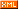# 大悟还俗

posts - 236, comments - 9, trackbacks - 0, articles - 0
::  ::::一、override  重载
type
TFigure = class
procedure Draw; virtual;//（我的理解是）父类中可以使用父类的，子类中使用子类的。与“四”是有区别的。
end;
TRectangle = class(TFigure)
procedure Draw; override;//a1
end;
TEllipse = class(TFigure)
procedure Draw; override;//a2
end;

var
Figure: TFigure;
begin
Figure := TRectangle.Create;
Figure.Draw;  // 执行a1
Figure.Destroy;
Figure := TEllipse.Create;
Figure.Draw;  // 执行a2
end;

type
T1 = class(TObject)
end;
T2 = class(T1)
end;
...
SomeObject := T2.Create;
SomeObject.Test('Hello!');  // T2.Test
SomeObject.Test(7);         // T1.Test

override是用来重载父类的procedure，

procedure DoSomething; virtual; abstract;//如果是这个样式的，表示要在子类中实现（代码）。而父类中，其他procedure function可以使用这个procedure。
（这里的virtual似乎不能被dynamic替换）。

2009-4-11

procedure DoSomething; virtual; abstract;
procedure DoSomething; dynamic; abstract;

if Assigned(DoSomething) then ...

type
TFigure = class
procedure Draw;
end;
TRectangle = class(TFigure)
procedure Draw;
end;
//实现
var
Figure: TFigure;
Rectangle: TRectangle;
begin
Figure := TFigure.Create;
Figure.Draw;  // 父类用父类的 TFigure.Draw
Figure.Destroy;
Figure := TRectangle.Create;
Figure.Draw;  // 虽然初始化为子类，但是由于申明的是父类，所以Figure还是调用了父类的Draw  TFigure.Draw
TRectangle(Figure).Draw;  // 强制转换成子类，就可以用TRectangle.Draw
Figure.Destroy;
Rectangle := TRectangle.Create;
Rectangle.Draw;  // 子类用子类的 TRectangle.Draw
Rectangle.Destroy;
end;

type
TFigure = class;  // forward declaration
TDrawing = class
Figure: TFigure;
...
end;
TFigure = class  // defining declaration
Drawing: TDrawing;
...
end;

2009-04-07

private
FItem:Integer
procedure SetItem(Value:Integer);virtual;//dynamic不允许
public
end;